Mathematics - Signals and Systems - Butterworth Filters[Image1]

Introduction

Hey it's a me again @drifter1!

Today we continue with my mathematics series about Signals and Systems to get into Butterworth Filters.

So, without further ado, let's dive straight into it!

Introduction to Butterworth Filters

Butterworth filters apply topics that we discussed in the previous article, which was about discrete-time system design. This useful class of filters is specified by two main parameters:

• Cut-off Frequency (ωc)
• Filter Order (N)
The filter order dictates the sharpness of the transaction from the pass-band to the stop-band. The frequency response is, thus, monotonic. When talking about continuous-time Butterworth filters, the poles are related to the square of the frequency response's magnitude. And in addition to that, they are distributed equally in angle on a circle in the s-plane, which is concentric to the origin and with a radius equal to the cut-off frequency. So, when knowing these two properties, the pole characteristics of the system function are also directly available. This also means, that the differential equation that describes the filter can also be obtained quite quickly.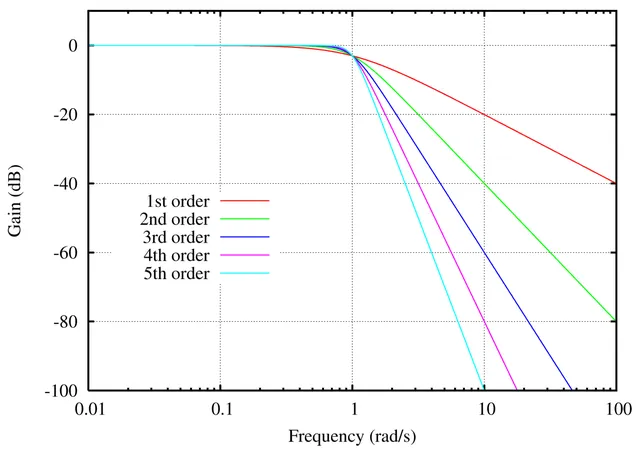[Image 2]

Applying techniques such as impulse invariance, it's possible to create discrete-time Butterworth filters. The filter specifications can be mapped to a corresponding set of specifications for the discrete-time filter, but there are of course some limitations. In order to avoid aliasing, the continuous-time filter needs to be band-limited. This can be done using a procedure known as bilinear transformation, where the entire imaginary axis in the s-plane is mapped around the unit circle. Since the imaginary axis is of infinite length, a non-linear distortion mapping between the two frequency axes occurs. Thus, even this technique can only be used when the filter is (at least) approximately a constant piecewise function (defined by cases).

Butterworth Filter Equation and Pole-Zero Plot

Mathematically, Butterworth filters are defined as: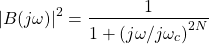In the s-plane, the square of the magnitude of the frequency response gives us:The poles of B(s) B(-s) are at: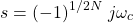which gives us pole-zero patterns of the following form: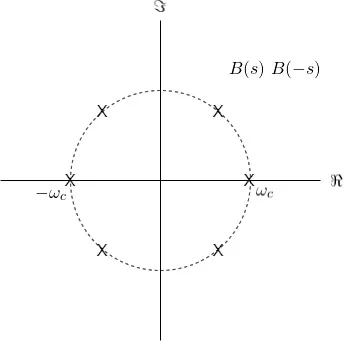Mapping using Impulse Invariance

Let's first talk about mapping the filter using Impulse Invariance. The sampled signal is taken as follows: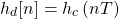As a Fourier series, the frequency response is defined as: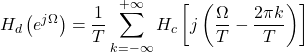For the Z-Transform, we need to determine B(s) and using partial fraction expansion we come up with:
Mapping using Bilinear Transformation

Using Bilinear Transformation, the following mapping occurs: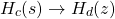where s and z are given by:For z = e , s is given by:Mapping from the continuous-time frequency axis (ω) to the discrete time frequency axis (Ω) is exactly what the Bilinear Transform is all about. So, in the end, it's basically the following mapping: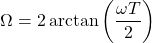RESOURCES:

Images

1.2.Block diagrams and other visualizations were made using draw.io

Final words | Next up

And this is actually it for today's post!

Next up is System Feedback...

See Ya!Keep on drifting!

H2
H3
H4
3 columns
2 columns
1 column
Join the conversation now